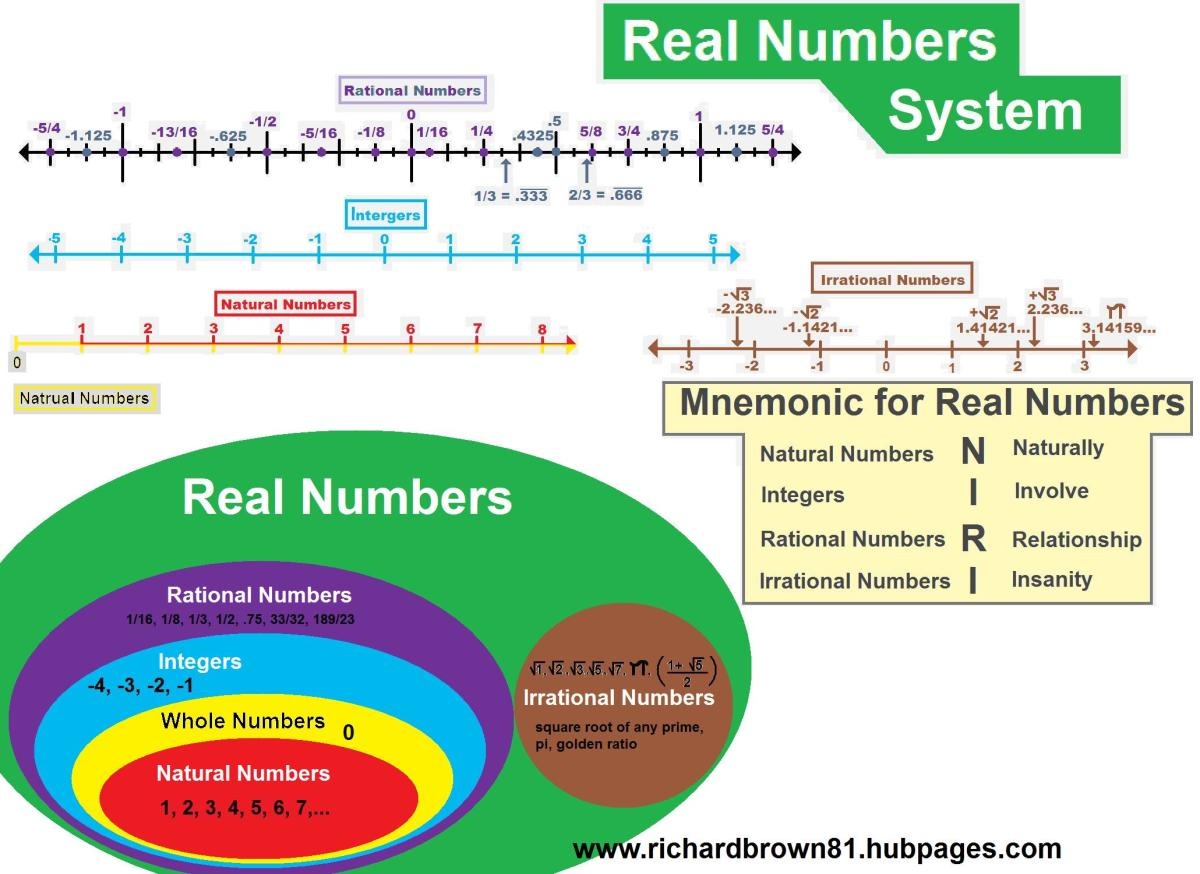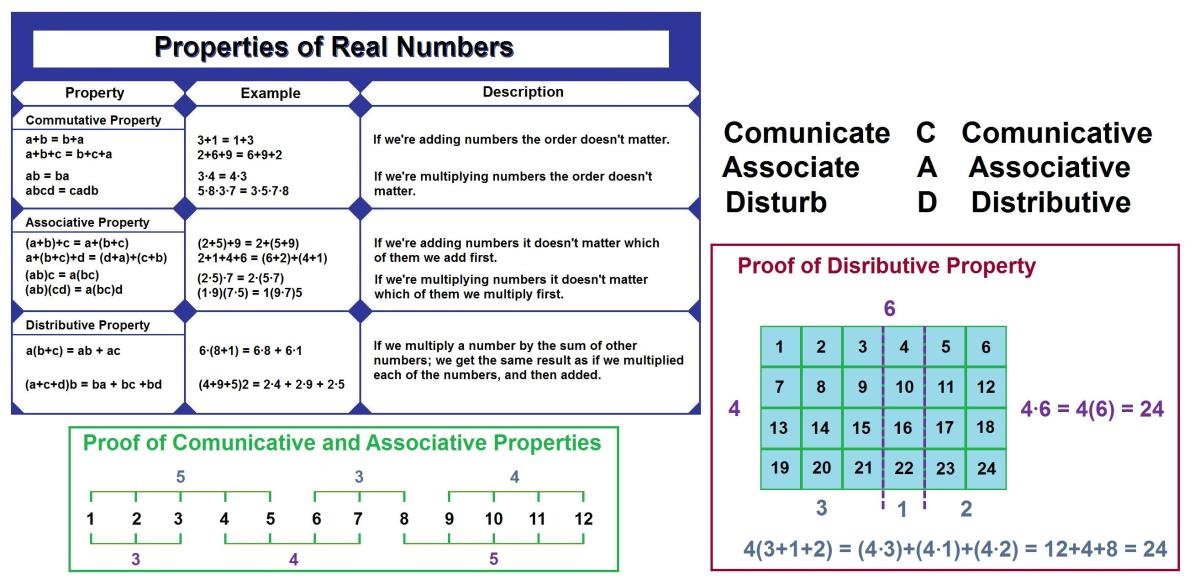# Real Numbers (Curious Concepts Precalculus 1.1)real number system, diagram, line dipictions, and mnemonic

## Self-explain Learning Technique

Self-explain Learning Technique helps people to better comprehend, and remember any thing they want to learn. It my sound strange, but it is many times more effective then re-reading. To use this technique, give short answers to the prompted questions. Incorporate previous knowledge-and-life experience into your answer will make this learning technique more effective. On average it improves learning by over 2.5 standard deviations; the difference between a C student and an A student.

How could knowing the properties of real numbers be helpful to you?

There are 4 types of real numers that make up the Real Number System: Natural, Integers, Rational, and Irrational. All of these have common properies that can be used to help solve problems.

Natural numbers are the first numbers you learned when you were a child. Remember counting beans; because you and all your classmates would have eaten the cand? These are Natural Numbers: 1, 2, 3, 4, 5, 6,... A number that you may have been familiar with, way back when, that isn't a natural number is 0. Zero is a weird number and until someone accually found a use for it, it was just a place holder (or a blank spot). Zero is not a real number, and an easy way to remember that is: you never learned to count by zeros.

Whole Numbers are all the Natural Numbers with the inclusion of 0.

Integers are the next ones you should have learned. Integers are like Whole Numbers, but the ones meaning less than 0 are negative. Whole Numbers are also integers. On a number line intergers are depicted in this order:

…, -4, -3, -2, -1, 0, 1, 2, 3, 4, ...

Rational Numbers are constructed by combining Integers in fractions.

…, -3/4, -5/8, -1/2, -3/8, -1/4, -1/8, 0, 1/8,1/4, 3/8, ½, 5/8, ¾, …

All Rational Numbers can be expressed as: r=m/n; were m and n are Intergers, and n does not equal 0.

Rational Numbers can be whole numbers, such as: 6/1 = 6

Rational numbers can also be expressed in decimal form, such as: 1/4 = 0.25

Examples of Rational Numbers are: 1/37, -5/17, 12 = 12/1, 0.24 = 24/100

Any problem were n equals 0 is undefined because you are trying to divide nothing into parts, and that's just non-sense. 4/0 is undefined because 4 can't be divided into 0 parts. 4 has to at least be divided into 1/∞ parts.

Irrational Numbers can't be expressed by a ratio of integers. The infinity symbol (∞) and many square roots of Natural Numbers are Irrational. The square root of any prime number can not be expressed as a ratio of integers. There is also the number = 3.14...., that many people are familiar with as the Circular Constant (pi). Irrational Numbers can represent m or n in r = m/n, making the ratio irrational.

Scroll to Continue

When the word number is used with out qualification, Real Number is implied.

Every Real Number has a Decimal Representation.

1234.987 has a 1 in the thousands place signifying that there is 1 thousand. There is a 2 in the hundreds place signifying 2 hundreds. There is a 3 in the tens place signifying 3 tens. There is a 4 in the ones place signifying 4 ones. Then we cross the decimal point, digits right the decimal signify that there is a continued value that is less then 1. There is a 9 in the tenths place, signifying that there is 9-1/10 or 9/10. There is an 8 in the hundredths place signifying 8-1/100 or 8/100. There is a 7 in the thousandths place signifying that there is 7-1/1000 or 7/1000. These all combine to create a single number. Each of the component numbers is called a digit. If we added each digit together we would get 1234.987.

Rational Numbers have decimals that are terminal or repeating: 1/8= 0.125000000... 1/3 = 0.3333333...

157/495 = 0.317171717... = 0.317 with a bar over the 17 indicating that the digits under it repeat forever.

Irrational Numbers do not have repeating decimals:

√2 = 1.41421356237... = 3.141592653589...

If we stop the representation decimal digits of any number (and round the final digit), we get an approximation. We use ≈ to show that it is not an exact equal: ≈ 3.1416. The more digits that are retained the the closer the approximation is.properies of real numbers with proof and mnemonic

## Self-Explain Learning Technique

How is it, that we can have different types of numbers?

## Properties of Real Numbers

In any pre-algebra class, we all learn that 2+3 = 3+2 and 5+3 = 3+5 and 124+3789 = 3789+124 and so on. We learn that we can express these infinite number of facts by writing their relationship.

a+b = b+a

Were a and b are any real number.

The order of addition doesn't matter because of the Commutative Propery of Addition. The commutative property holds true for multiplication. We can multiply in any order and get the same answer.

The Associative Property states that it doesn't matter which terms we add together first; we still get the same answer. We can add 5 and 3, then add another 12; or we can add 5 and 12 then 3. We will get the same answer, no matter how many or which numbers, are added first. This is true for addition and multiplication also, we can multiply any amount of number in any order and get the same answer every time.

The Distributive Property is were thing stat to get interesting. It shows how addition and multiplication interact. It holds true for all real numbers.